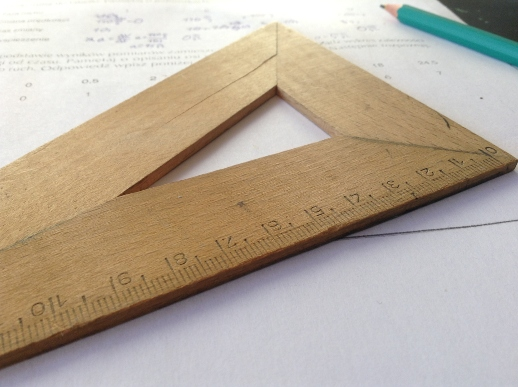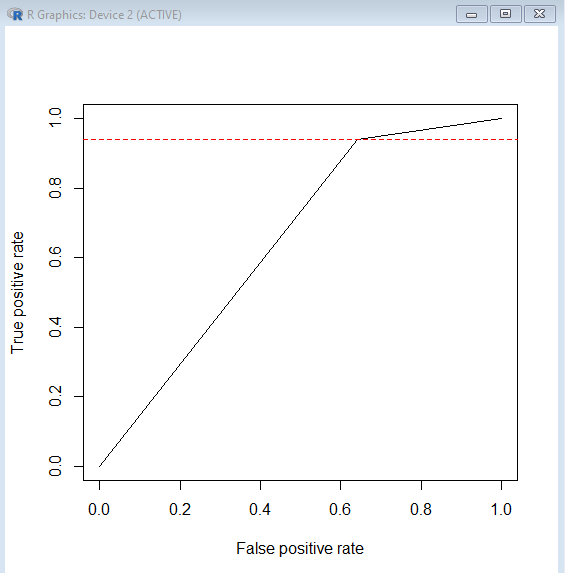{{announcement.body}}
{{announcement.title}}

# Breezing Through Support Vector Machines

DZone 's Guide to

# Breezing Through Support Vector Machines

### In this article, we discuss some basics behind how vector algebra works under-the-hood in machine learning models.

· Big Data Zone ·
Free Resource

Comment (0)

Save
{{ articles.views | formatCount}} ViewsWhen we add "Machine" to anything it looks cool... perhaps due to an assumption made about the introduction of both "intelligence" and "automation" hinted by the use of such a term.

So, let's take that out and we are back to old, classical vector algebra. It's like a person with a bunch of sticks to figure out which one to lay where in a 2-D plane to separate one class of objects from another, provided class definitions are already known.

The problem is which particular shape and length must be chosen to show maximum contrast between classes.

We need to arrive at a function definition, in such a way that the value a given function takes changes drastically (e.g. from a large positive value to a large negative value).

In it's simplest form, output Y can be represented as a vector product of two independent vectors K and X respectively, where we have to compute values of vector K, given input vector X, so that it minimizes the error between computed approximation Yhat and Y.

R

`x`

1
`Yhat<-t(K)*X`

You may also like: R Essentials.

(K)*(X) is the generalized dot product (a.k.a. the "inner product" of input vectors). Doing the dot product of  X with transpose of K is equivalent to computing the projection of vector X on vector K. This is the "shadow" cast of one vector on another.

To put it more simply, it is a weighted sum of values of vectors K and X respectively; for each observation value of X, where the corresponding value of K for a given value of X must be computed in order to arrive at Yhat, which most closely resembles the classification sets that exist in Y.

R

`xxxxxxxxxx`
1

1
`K1*X1+K2*X2+K3*X3+...KN*XN`

The value that such computation takes, considering that it will be a numeric value, must provide a  good enough demarcation in order to separate different classes that exist in Y.  Such "demarcation"  has to be as wide as possible so as not to interfere with individual class boundaries.

The contour of such demarcation depends on the dimensional space we are operating in.

For a 2-D space, it is simply a plane ( Z1*X+Z2*Y=C) — think about a DMZ zone or a "No man's land" that exists between nations that share borders. And this plane would evaluate to a starkly contrasting value (-C for one side and +C for another, for a symmetrical demarcation to exist between the two class boundaries).

In fact, the equation Yhat<-t(K)*X , which is same as : K1*X1+K2*X2+K3*X3+...KN*XN, for N observations, is indeed this plane that seeks to distinguish and separate the two classes on either side.

A starker contrast in computed value on either side of demarcation, will result in more pronounced errors of misclassification and in turn enable optimization algorithm to minimize such errors by adjusting values of vector K.

R

`xxxxxxxxxx`
1

1
`K1*X1+K2*X2= -100 for class A`
2
`K1*X1 +K2*X2 =+100 for class B`
3

As an example, the computed value abruptly switches from -100 to +100, as the model tries to distinguish Class A from Class B.

The optimization objective for the algorithm would be to maximize this abrupt contrast.

Obviously, the planes can distinguish linearly distributed classes. If we can't imagine a line that can separate the two classes without bending or twisting it, then we have look at non-linear solutions to arriving at providing maximum contrast.

The problem is similar to taking a stroll or drive to remember important landmarks so that we can make it back or go there again. In doing so, every location that one visits needs to be compared in similarity with a set of previously identified landmarks.

Hence, we need a similarity function that tells in Binary or Boolean terms as to whether a given observation is very similar to a given landmark.

Similarity functions can be generally expressed as Similarity(L, X).

One commonly used form would be a polynomial expressed as:

R

`x`

1
`((gamma*t(x))*L+c)^a ##where epsilon, c are constants to make coefficients of vector product more generic and open for selection appropriate for optimization, while a is an integer. gamma >0`

Another popular, general function can be Gaussian — one that takes the form:

R

`xxxxxxxxxx`
1

1
`exp(-Z^2/2) ##where Z= (x[i]-mean(x))/sd(x)`

We are tweaking it by replacing mean(X) by LM[i], where LM is a vector of Landmarks. Then, we introduce constant epsilon (just like we did in polynomial similarity function), which in this case takes a value as (1/(2*sd(x)^2))

R

`x`

1
`exp(-gamma*(K^2)) ##where K= (x[i]-LM[i]) and gamma is a constant= (1/(2*(sd(x))^2))`

Obviously, it can be hard to choose which similarity function would be most suitable and what should be the values of various constants.

In R, the function `best.svm` comes in handy (in package e1071).

We will use this on our previous data set german_credit.csv. ( Refer to my previous article.)

R

`xxxxxxxxxx`
1
21

1
` #tune and decide on SVM parameters`
2
`> `
3
`> best.svm(Creditability~., y = NULL, data = train, degree = NULL, gamma = NULL, coef0 = NULL,`
4
`+          cost = NULL, nu = NULL, class.weights = NULL, epsilon = NULL, kernel="radial")`
5

6
`Call:`
7
`best.svm(x = Creditability ~ ., y = NULL, data = train, degree = NULL, `
8
`    gamma = NULL, coef0 = NULL, cost = NULL, nu = NULL, class.weights = NULL, `
9
`    epsilon = NULL, kernel = "radial")`
10

11

12
`Parameters:`
13
`   SVM-Type:  eps-regression `
14
` SVM-Kernel:  radial `
15
`       cost:  1 `
16
`      gamma:  0.05 `
17
`    epsilon:  0.1 `
18

19

20
`Number of Support Vectors:  379`
21

`best.svm` has now automatically selected the similarity function type as Radial. (Similar to the Gaussian function definition, as discussed here).

R

`xxxxxxxxxx`
1

1
`model<-svm(as.factor(Creditability)~.,data=train,cost=1,gamma=0.05, epsilon=0.1,kernel="radial")`
2

R

`xxxxxxxxxx`
1
30

1
`## 1.predict using model , then 2.populate the performance object in ROCR package and now 3. plot confusion matrix to observe various performance metrics and 4. plot visually using npr as elbow line.`
2
`confusionMatrix(as.factor(dfcomp\$Creditability),as.factor(dfcomp\$svm))`
3
`Confusion Matrix and Statistics`
4

5
`          Reference`
6
`Prediction   0   1`
7
`         0  70 125`
8
`         1  25 388`
9
`                                         `
10
`               Accuracy : 0.7533         `
11
`                 95% CI : (0.717, 0.7871)`
12
`    No Information Rate : 0.8438         `
13
`    P-Value [Acc > NIR] : 1              `
14
`                                         `
15
`                  Kappa : 0.3452         `
16
` Mcnemar's Test P-Value : 6.303e-16      `
17
`                                         `
18
`            Sensitivity : 0.7368         `
19
`            Specificity : 0.7563         `
20
`         Pos Pred Value : 0.3590         `
21
`         Neg Pred Value : 0.9395         `
22
`             Prevalence : 0.1562         `
23
`         Detection Rate : 0.1151         `
24
`   Detection Prevalence : 0.3207         `
25
`      Balanced Accuracy : 0.7466         `
26
`                                         `
27
`       'Positive' Class : 0  `
28

29
`plot(perf2)`
30
`abline(h=0.9395,col="RED",lty=2)`
31Topics:
support vector machines ,big data ,r ,rhodium export statistics ,machine learning ,tensorflow ,tutorial

Comment (0)

Save
{{ articles.views | formatCount}} Views

Opinions expressed by DZone contributors are their own.